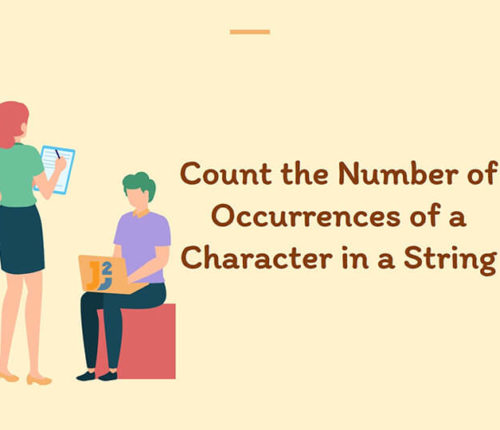# Java program to find first and last digit of a number

In this article, we are going to find first and last digit of a number in Java.
To find first and last digit of any number, we can have several ways like using modulo operator or pow() and `log()` methods of `Math` class etc.

Let’s see some examples.
Before moving to example, let’s first create an algorithm to find first and last digit of a number.

## Algorithm

`Step 1:` Take a number from the user.
`Step 2:` Create two variables `firstDigit` and `lastDigit` and initialize them by 0.
`Step 3:` Use modulo operator(`%`) to find last digit.
`Step 4:` Use either while loop and division operator (`/`) or `log()` and `pow()` methods of `Math` class to find

.
`Step 5:` Display the result.

### Using while loop

It is easy to use modulo operator that will return the last digit of the number, and we can use while loop and division operator to get first digit of number.

Output

Last digit: 7
First digit: 5

### Using log() and pow() methods

If we want to use `built-in methods` like log() and pow() of Math class, then we can use `log()` method to get the number of digits and `pow()` method to get divisor value that later on used to get the first digit of number. See the example below.

Output

Last digit: 7
First digit: 5

### Using while loop and pow() method

If we don’t want to use `log()` method then use while loop to count the number of digits and use that count into the pow() method to get divisor. See the example below.

Output:

Last digit: 7
First digit: 5

That’s all about Java program to find first and last digit of number in java.

import_contacts

### You may also like:## Related Posts

•05 April

### Count occurrences of Character in String in Java

Table of Contents1. Using String Library Methods2. Using Recursion3. Using Hashing ConceptUsing ArraysUsing Collections (Map) 4. Using Java 8 Features In this article, we will look at a problem: Given an Input String and a Character, we have to Count Occurrences Of character in String in java. For Example, If the Given String is : […]

•24 December

### How to capitalize first letter in java

Learn about how to capitalize first letter in java.

•03 October

### Happy Number program in Java

Table of ContentsWhat is a Happy Number?Using HashsetAlgorithmExampleUsing slow and fast pointersAlgorithmExample In this article, we are going to learn to find Happy Number using Java. Let’s first understand, what is Happy Number? What is a Happy Number? A number which leaves 1 as a result after a sequence of steps and in each step […]

•30 September

### Find Perfect Number in Java

Table of ContentsIterative approachRecursive approach In this article, we are going to find whether a number is perfect or not using Java. A number is called a perfect number if the sum of its divisors is equal to the number. The sum of divisors excludes the number. There may be several approaches to find the […]

•28 September

### How to find Magic Number in Java

Table of ContentsWhat is a Magic Number?Algorithm for Magic NumberExample to find Magic NumberAnother Example To find Magic Number In this article, we are going to learn to find Magic Number using Java. Let’s first understand, what is Magic Number? What is a Magic Number? A number which leaves 1 as a result after a […]

•28 September

### Number guessing game in java

Table of ContentsNumber guessing game RulesAlgorithm for Number guessing game In this article, we will implement Number guessing game in java. The number guessing game is based on a concept where player guesses a number between a range. If player guesses the exact number then player wins else player looses the game. Since this game […]

## Subscribe to our newletter

Get quality tutorials to your inbox. Subscribe now.